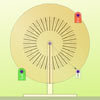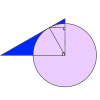Search by Topic

Resources tagged with Sine, cosine, tangent similar to Complex Sine:

Filter by: Content type:
Age range:
Challenge level:

There are 59 results

Broad Topics > Pythagoras and Trigonometry > Sine, cosine, tangentPumping the Power

Age 16 to 18 Challenge Level:

What is an AC voltage? How much power does an AC power source supply?Eight Ratios

Age 14 to 16 Challenge Level:

Two perpendicular lines lie across each other and the end points are joined to form a quadrilateral. Eight ratios are defined, three are given but five need to be found.Bend

Age 16 to 18 Challenge Level:

What is the longest stick that can be carried horizontally along a narrow corridor and around a right-angled bend?Far Horizon

Age 14 to 16 Challenge Level:

An observer is on top of a lighthouse. How far from the foot of the lighthouse is the horizon that the observer can see?Trigonometric Protractor

Age 14 to 16 Challenge Level:

An environment that simulates a protractor carrying a right- angled triangle of unit hypotenuse.Coke Machine

Age 14 to 16 Challenge Level:

The coke machine in college takes 50 pence pieces. It also takes a certain foreign coin of traditional design...Ball Bearings

Age 16 to 18 Challenge Level:

If a is the radius of the axle, b the radius of each ball-bearing, and c the radius of the hub, why does the number of ball bearings n determine the ratio c/a? Find a formula for c/a in terms of n.Orbiting Billiard Balls

Age 14 to 16 Challenge Level:

What angle is needed for a ball to do a circuit of the billiard table and then pass through its original position?Three by One

Age 16 to 18 Challenge Level:

There are many different methods to solve this geometrical problem - how many can you find?30-60-90 Polypuzzle

Age 16 to 18 Challenge Level:

Re-arrange the pieces of the puzzle to form a rectangle and then to form an equilateral triangle. Calculate the angles and lengths.8 Methods for Three by One

Age 14 to 18 Challenge Level:

This problem in geometry has been solved in no less than EIGHT ways by a pair of students. How would you solve it? How many of their solutions can you follow? How are they the same or different?. . . .Moving Squares

Age 14 to 16 Challenge Level:

How can you represent the curvature of a cylinder on a flat piece of paper?Age 14 to 16 Challenge Level:

In this problem we are faced with an apparently easy area problem, but it has gone horribly wrong! What happened?Circle Scaling

Age 14 to 16 Challenge Level:

Describe how to construct three circles which have areas in the ratio 1:2:3.Shape and Territory

Age 16 to 18 Challenge Level:

If for any triangle ABC tan(A - B) + tan(B - C) + tan(C - A) = 0 what can you say about the triangle?Round and Round a Circle

Age 14 to 16 Challenge Level:

Can you explain what is happening and account for the values being displayed?Small Steps

Age 16 to 18 Challenge Level:

Two problems about infinite processes where smaller and smaller steps are taken and you have to discover what happens in the limit.Screen Shot

Age 14 to 16 Challenge Level:

A moveable screen slides along a mirrored corridor towards a centrally placed light source. A ray of light from that source is directed towards a wall of the corridor, which it strikes at 45 degrees. . . .Six Discs

Age 14 to 16 Challenge Level:

Six circular discs are packed in different-shaped boxes so that the discs touch their neighbours and the sides of the box. Can you put the boxes in order according to the areas of their bases?Sine and Cosine

Age 14 to 16 Challenge Level:

The sine of an angle is equal to the cosine of its complement. Can you explain why and does this rule extend beyond angles of 90 degrees?Spokes

Age 16 to 18 Challenge Level:

Draw three equal line segments in a unit circle to divide the circle into four parts of equal area.Logosquares

Age 16 to 18 Challenge Level:

Ten squares form regular rings either with adjacent or opposite vertices touching. Calculate the inner and outer radii of the rings that surround the squares.Inscribed in a Circle

Age 14 to 16 Challenge Level:

The area of a square inscribed in a circle with a unit radius is, satisfyingly, 2. What is the area of a regular hexagon inscribed in a circle with a unit radius?Raising the Roof

Age 14 to 16 Challenge Level:

How far should the roof overhang to shade windows from the mid-day sun?Circle Box

Age 14 to 16 Challenge Level:

It is obvious that we can fit four circles of diameter 1 unit in a square of side 2 without overlapping. What is the smallest square into which we can fit 3 circles of diameter 1 unit?Age 14 to 16 Challenge Level:

If you were to set the X weight to 2 what do you think the angle might be?Round and Round

Age 14 to 16 Challenge Level:

Prove that the shaded area of the semicircle is equal to the area of the inner circle.Pythagoras on a Sphere

Age 16 to 18 Challenge Level:

Prove Pythagoras' Theorem for right-angled spherical triangles.Why Stop at Three by One

Age 16 to 18

Beautiful mathematics. Two 18 year old students gave eight different proofs of one result then generalised it from the 3 by 1 case to the n by 1 case and proved the general result.A Scale for the Solar System

Age 14 to 16 Challenge Level:

The Earth is further from the Sun than Venus, but how much further? Twice as far? Ten times?History of Trigonometry - Part 3

Age 11 to 18

The third of three articles on the History of Trigonometry.Farhan's Poor Square

Age 14 to 16 Challenge Level:

From the measurements and the clue given find the area of the square that is not covered by the triangle and the circle.After Thought

Age 16 to 18 Challenge Level:

Which is larger cos(sin x) or sin(cos x) ? Does this depend on x ?Cosines Rule

Age 14 to 16 Challenge Level:

Three points A, B and C lie in this order on a line, and P is any point in the plane. Use the Cosine Rule to prove the following statement.History of Trigonometry - Part 2

Age 11 to 18

The second of three articles on the History of Trigonometry.Diagonals for Area

Age 16 to 18 Challenge Level:

Can you prove this formula for finding the area of a quadrilateral from its diagonals?The History of Trigonometry- Part 1

Age 11 to 18

The first of three articles on the History of Trigonometry. This takes us from the Egyptians to early work on trigonometry in China.Geometric Trig

Age 16 to 18 Short Challenge Level:

Trigonometry, circles and triangles combine in this short challenge.At a Glance

Age 14 to 16 Challenge Level:

The area of a regular pentagon looks about twice as a big as the pentangle star drawn within it. Is it?Age 14 to 16 Challenge Level:

The sides of a triangle are 25, 39 and 40 units of length. Find the diameter of the circumscribed circle.Squ-areas

Age 14 to 16 Challenge Level:

Three squares are drawn on the sides of a triangle ABC. Their areas are respectively 18 000, 20 000 and 26 000 square centimetres. If the outer vertices of the squares are joined, three more. . . .Muggles, Logo and Gradients

Age 11 to 18

Logo helps us to understand gradients of lines and why Muggles Magic is not magic but mathematics. See the problem Muggles magic.Figure of Eight

Age 14 to 16 Challenge Level:

On a nine-point pegboard a band is stretched over 4 pegs in a "figure of 8" arrangement. How many different "figure of 8" arrangements can be made ?Sine and Cosine for Connected Angles

Age 14 to 16 Challenge Level:

The length AM can be calculated using trigonometry in two different ways. Create this pair of equivalent calculations for different peg boards, notice a general result, and account for it.Strange Rectangle 2

Age 16 to 18 Challenge Level:

Find the exact values of some trig. ratios from this rectangle in which a cyclic quadrilateral cuts off four right angled triangles.Sine Problem

Age 16 to 18 Challenge Level:

In this 'mesh' of sine graphs, one of the graphs is the graph of the sine function. Find the equations of the other graphs to reproduce the pattern.Dodecawhat

Age 14 to 16 Challenge Level:

Follow instructions to fold sheets of A4 paper into pentagons and assemble them to form a dodecahedron. Calculate the error in the angle of the not perfectly regular pentagons you make.Octa-flower

Age 16 to 18 Challenge Level:

Join some regular octahedra, face touching face and one vertex of each meeting at a point. How many octahedra can you fit around this point?Trig Reps

Age 16 to 18 Challenge Level:

Can you deduce the familiar properties of the sine and cosine functions starting from these three different mathematical representations?From All Corners

Age 14 to 16 Challenge Level:

Straight lines are drawn from each corner of a square to the mid points of the opposite sides. Express the area of the octagon that is formed at the centre as a fraction of the area of the square.